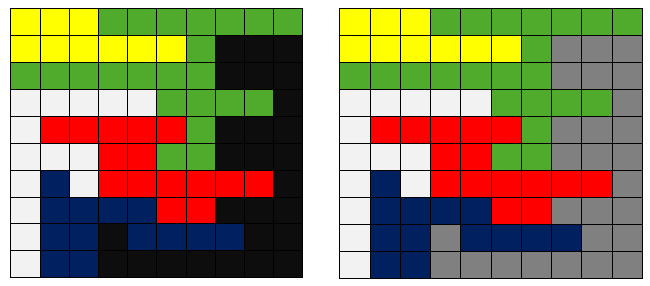# Flood Fill Algorithm

Flood fill (also known as seed fill) is an algorithm that determines the area connected to a given node in a multi-dimensional array.

It is used in the “bucket” fill tool of paint program to fill connected, similarly-colored areas with a different color, and in games such as Go and Minesweeper for determining which pieces are cleared. When applied on an image to fill a particular bounded area with color, it is also known as boundary fill.

The flood-fill algorithm takes three parameters: a start node, a target color, and a replacement color. Consider below matrix to the left – if start node = (3, 9), target color = "BLACK" and replacement color = "GREY", the algorithm looks for all nodes in the matrix that are connected to the start node by a path of the target color and changes them to the replacement color.Note that each cell of the matrix represents one pixel.

##### Approach 1: (Using BFS)

An explicitly queue-based implementation is shown in pseudo-code below.

BFS (starting-pixel, replacement-color)

1. Create an empty queue

2. Enqueue starting pixel and mark it as processed

3. do till queue is not empty

a) Pop front node from the queue and process it

b) Replace color of current pixel (popped node) with that of replacement

c) Process all 8 adjacent pixels of current pixel and enqueue each valid
pixel which has same color as that of current pixel

## C++

Output:

Y Y Y G G G G G G G
Y Y Y Y Y Y G C C C
G G G G G G G C C C
W W W W W G G G G C
W R R R R R G C C C
W W W R R G G C C C
W B W R R R R R R C
W B B B B R R C C C
W B B C B B B B C C
W B B C C C C C C C

## Java

Output:

[Y, Y, Y, G, G, G, G, G, G, G]
[Y, Y, Y, Y, Y, Y, G, C, C, C]
[G, G, G, G, G, G, G, C, C, C]
[W, W, W, W, W, G, G, G, G, C]
[W, R, R, R, R, R, G, C, C, C]
[W, W, W, R, R, G, G, C, C, C]
[W, B, W, R, R, R, R, R, R, C]
[W, B, B, B, B, R, R, C, C, C]
[W, B, B, C, B, B, B, B, C, C]
[W, B, B, C, C, C, C, C, C, C]

Time complexity of above solution is O(MN).

##### Approach 2: (Using DFS)

We can use DFS to solve this problem. The idea is to start from the source node in the matrix, replace its color with replacement color and recursively explore all its valid eight adjacent pixels and replace their color as well. Note that we don’t need a visited array here as we are replacing the color of every processed node and it won’t be considered again next time as it will have a different color.

## C++

Output:

Y Y Y G G G G G G G
Y Y Y Y Y Y G C C C
G G G G G G G C C C
W W W W W G G G G C
W R R R R R G C C C
W W W R R G G C C C
W B W R R R R R R C
W B B B B R R C C C
W B B C B B B B C C
W B B C C C C C C C

## Java

Output:

[Y, Y, Y, G, G, G, G, G, G, G]
[Y, Y, Y, Y, Y, Y, G, C, C, C]
[G, G, G, G, G, G, G, C, C, C]
[W, W, W, W, W, G, G, G, G, C]
[W, R, R, R, R, R, G, C, C, C]
[W, W, W, R, R, G, G, C, C, C]
[W, B, W, R, R, R, R, R, R, C]
[W, B, B, B, B, R, R, C, C, C]
[W, B, B, C, B, B, B, B, C, C]
[W, B, B, C, C, C, C, C, C, C]

Time complexity of above solution is O(MN).

References: Flood Fill – Wikipedia(1 votes, average: 5.00 out of 5)Loading...

Please use our online compiler to post code in comments. To contribute, get in touch with us.
Like us? Please spread the word and help us grow. Happy coding 🙂Subscribe
Notify ofGuest
carlochess

I don’t think that you need the processed matrix in the first solution:
http://ideone.com/uRjayDGuest

I think you need to check the processed on the first example. Check if processed[x+row[k]][y+col[k]] is false.